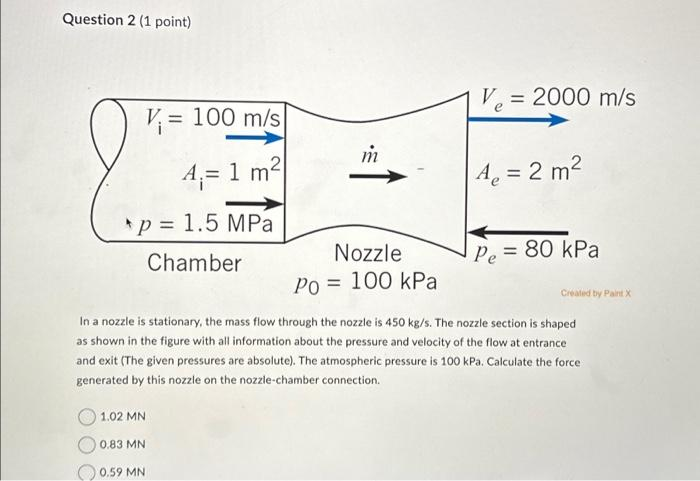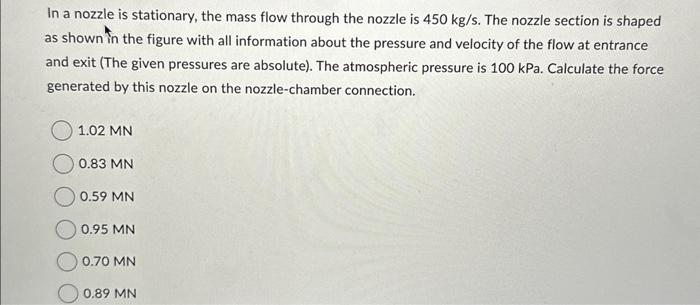# (Solved): Question 2 (1 point) V. V = 100 m/s A= 1 m * p = 1.5 MPa Chamber 1.02 MN 0.83 MN m 0.59 MN N ...

Question 2 (1 point) V. V? = 100 m/s A?= 1 m² * p = 1.5 MPa Chamber 1.02 MN 0.83 MN m 0.59 MN Nozzle Po = 100 kPa V? = 2000 m/s e A? = 2 m² Ae In a nozzle is stationary, the mass flow through the nozzle is 450 kg/s. The nozzle section is shaped as shown in the figure with all information about the pressure and velocity of the flow at entrance and exit (The given pressures are absolute). The atmospheric pressure is 100 kPa. Calculate the force generated by this nozzle on the nozzle-chamber connection. Pe = 80 kPa Created by Paint XQuestion 2 (1 point) In a nozzle is stationary, the mass flow through the nozzle is . The nozzle section is shaped as shown in the figure with all information about the pressure and velocity of the flow at entrance and exit (The given pressures are absolute). The atmospheric pressure is . Calculate the force generated by this nozzle on the nozzle-chamber connection. In a nozzle is stationary, the mass flow through the nozzle is . The nozzle section is shaped as shown in the figure with all information about the pressure and velocity of the flow at entrance and exit (The given pressures are absolute). The atmospheric pressure is . Calculate the force generated by this nozzle on the nozzle-chamber connection.

We have an Answer from Expert